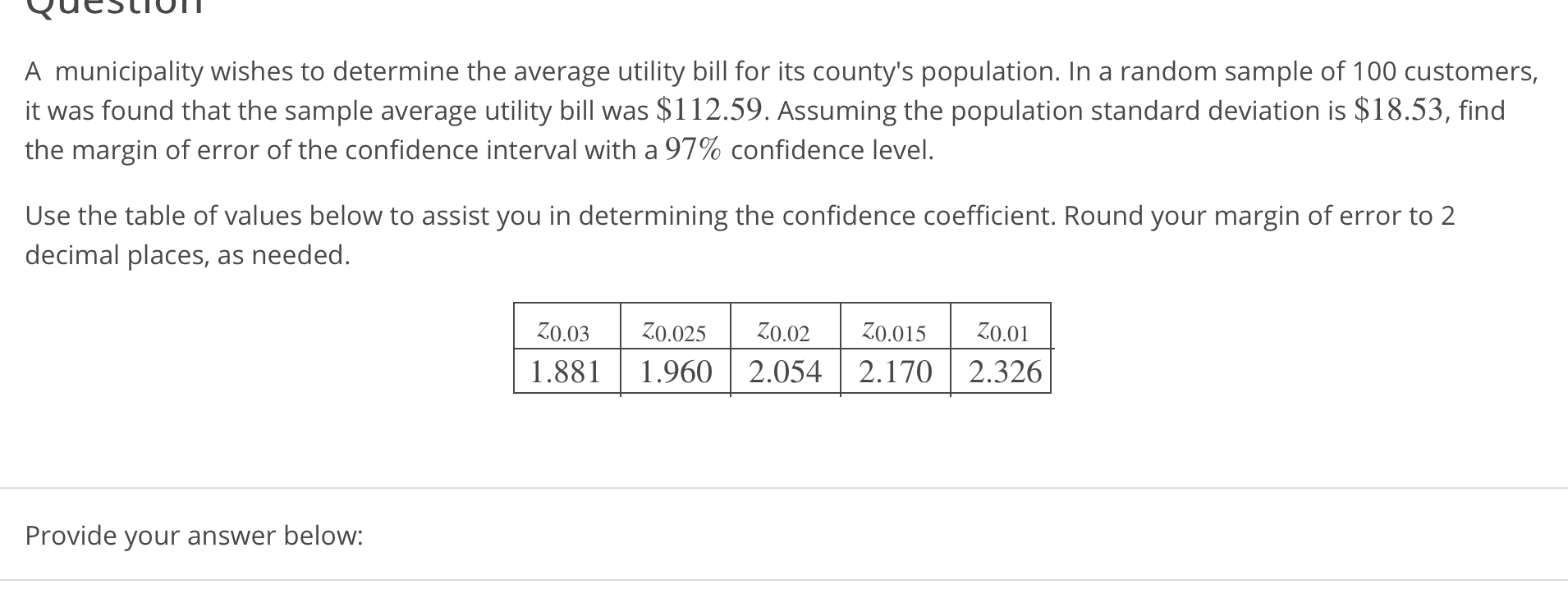# A municipality wishes to determine the average utility bill for its county's population. In a random sample of 100 customers,it was found that the sample average utility bill was \$112.59. Assuming the population standard deviation is \$18.53, findthe margin of error of the confidence interval with a 97% confidence level.Use the table of values below to assist you in determining the confidence coefficient. Round your margin of error to 2decimal places, as needed.1.881 1.9602.0542.170 2.326Provide your answer below:

Questionhelp_outlineImage TranscriptioncloseA municipality wishes to determine the average utility bill for its county's population. In a random sample of 100 customers, it was found that the sample average utility bill was \$112.59. Assuming the population standard deviation is \$18.53, find the margin of error of the confidence interval with a 97% confidence level. Use the table of values below to assist you in determining the confidence coefficient. Round your margin of error to 2 decimal places, as needed. 1.881 1.9602.0542.170 2.326 Provide your answer below: fullscreen
check_circleExpert Solution
Step 1

Margin of error:

Margin of error is a range of values that lie above and below the sample statistic of the confidence interval; it gives the percentage of number of points that differ in the obtained results from the population value.

Margin of error is calculated as the product of critical value and standard deviation or the product of critical value and standard error of the statistic.

The general formula for obtaining margin of error is,

Step 2

Obtain the critical value:

The required confidence level is 100*(1–α)% = 97%.

The required conditions for using standard normal distribution to estimate the confidence interval about mean is given below:

• The population standard deviation σ must be known.
• Either the sample size (n) must be greater than 30 or the population must be normally distributed.

Here, it is given that the sample size is 100 (which is greater than 30) and the population standard deviation is known.

From the given common z-values using standard normal distribution the critical value is obtained as 2.17 from the calculation given below:

Step 3

Find the margin of error of the confidence interval with 97% confidence level:

Given that, the population standard deviation is σ = 18.53,

The sample mean is x-bar = 112.59,

The sample size is n = 100,

The critical value is z(α/2) = z...

### Want to see the full answer?

See Solution

#### Want to see this answer and more?

Solutions are written by subject experts who are available 24/7. Questions are typically answered within 1 hour*

See Solution
*Response times may vary by subject and question
Tagged in

### Hypothesis Testing